# How to Calculate and Solve for Linear Momentum | Motion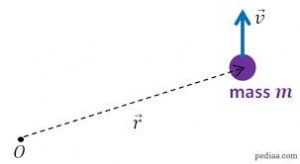The image above represents linear momentum.

To compute for linear momentum, two essential parameters are needed and these parameters are mass (m) and velocity (v).

The formula for calculating linear momentum:

p = mv

Where;

p = Momentum
m = Mass
v = Velocity

Let’s solve an example;
Find the momentum when the mass is 8 and the velocity is 12.

This implies that;

m = Mass = 8
v = Velocity = 12

p = mv
p = 8 x 12
p = 96

Therefore, the momentum is 96 Kgm/s.

Calculating the Mass when the Momentum and the Velocity is Given.

m = p / v

Where;

m = Mass
p = Momentum
v = Velocity

Let’s solve an example;
Find the mass when the momentum is 40 and the velocity is 18.

This implies that;

p = Momentum = 40
v = Velocity = 18

m = p / v
m = 40 / 18
m = 2.22

Therefore, the mass is 2.22 kg.

Calculating the Velocity when the Momentum and the Mass is Given.

v = p / m

Where;

v = Velocity
p = Momentum
m = Mass

Let’s solve an example;
Find the velocity when the momentum is 35 and the mass is 10.

This implies that;

p = Momentum = 35
m = Mass = 10

v = p / m
v = 35 / 10
v = 3.5

Therefore, the velocity is 3.5 m/s.

Nickzom Calculator – The Calculator Encyclopedia is capable of calculating the linear momentum.

To get the answer and workings of the linear momentum using the Nickzom Calculator – The Calculator Encyclopedia. First, you need to obtain the app.

You can get this app via any of these means:

To get access to the professional version via web, you need to register and subscribe for NGN 1,500 per annum to have utter access to all functionalities.
You can also try the demo version via https://www.nickzom.org/calculator

Apple (Paid) – https://itunes.apple.com/us/app/nickzom-calculator/id1331162702?mt=8
Once, you have obtained the calculator encyclopedia app, proceed to the Calculator Map, then click on Motion under Physics.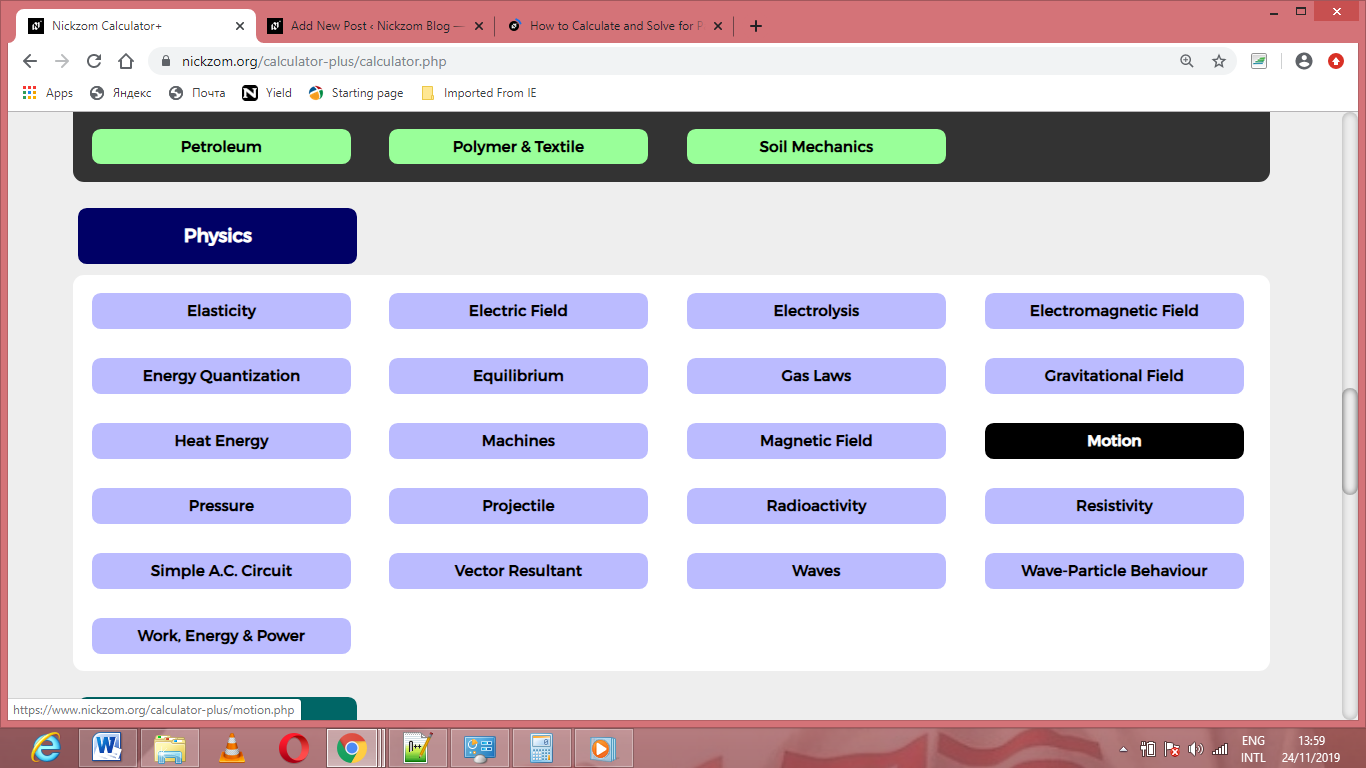Now, Click on Linear Momentum under Motion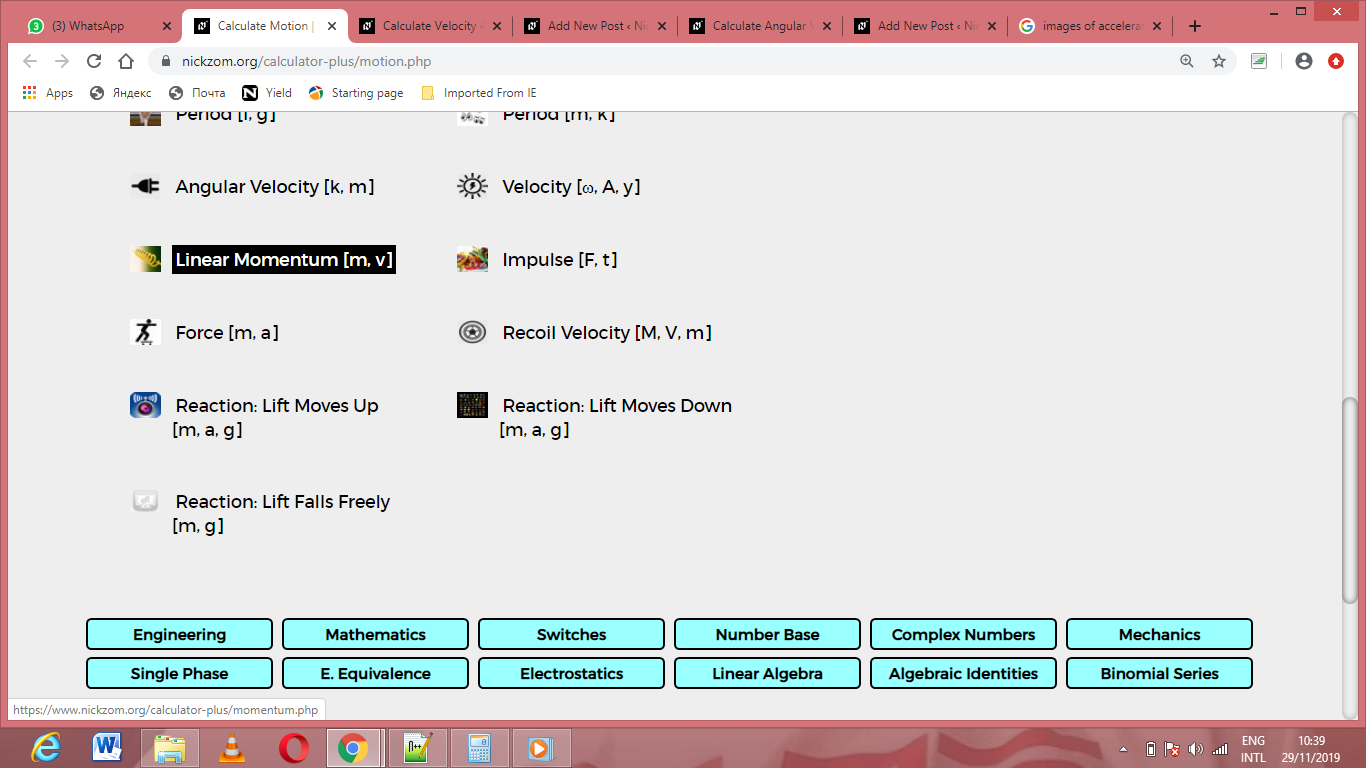The screenshot below displays the page or activity to enter your values, to get the answer for the linear momentum according to the respective parameters which are the mass (m) and velocity (v).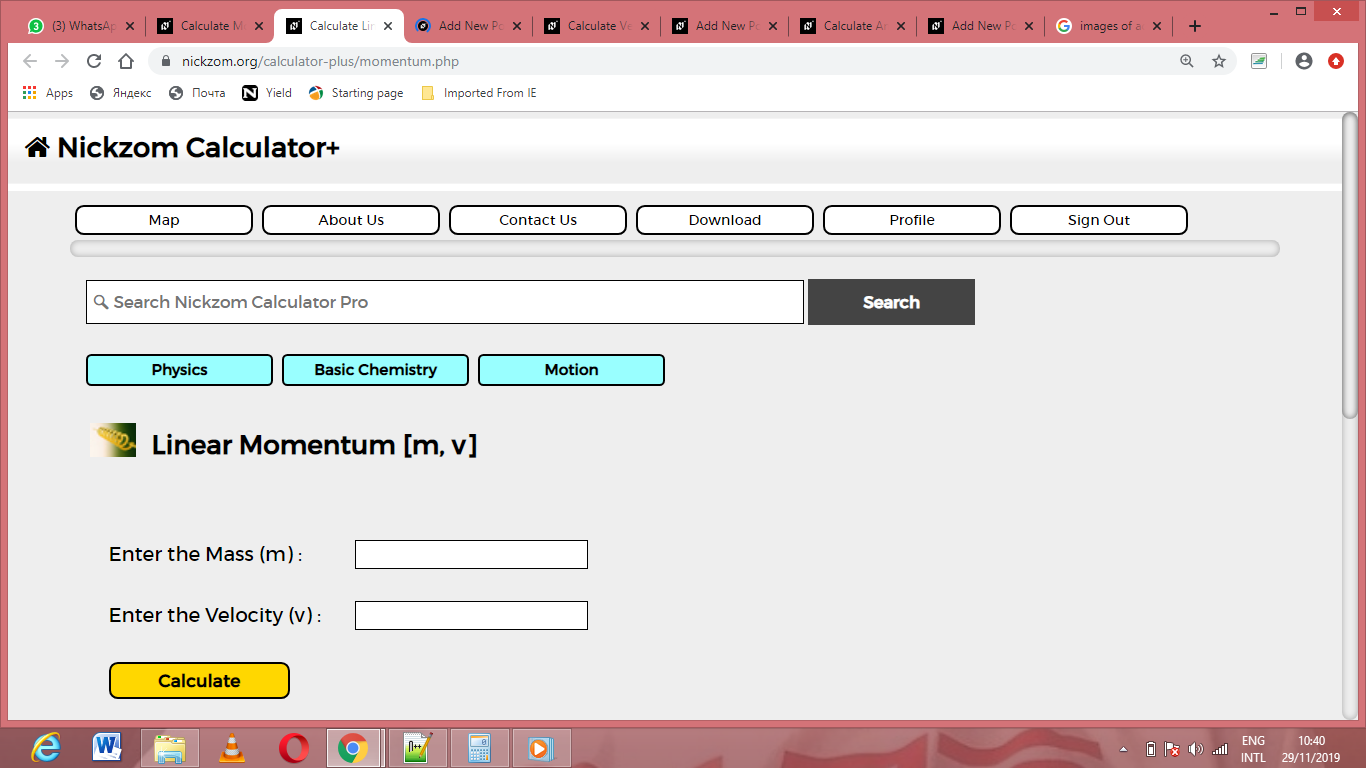Now, enter the values appropriately and accordingly for the parameters as required by the mass (m) is 8 and velocity (v) is 12.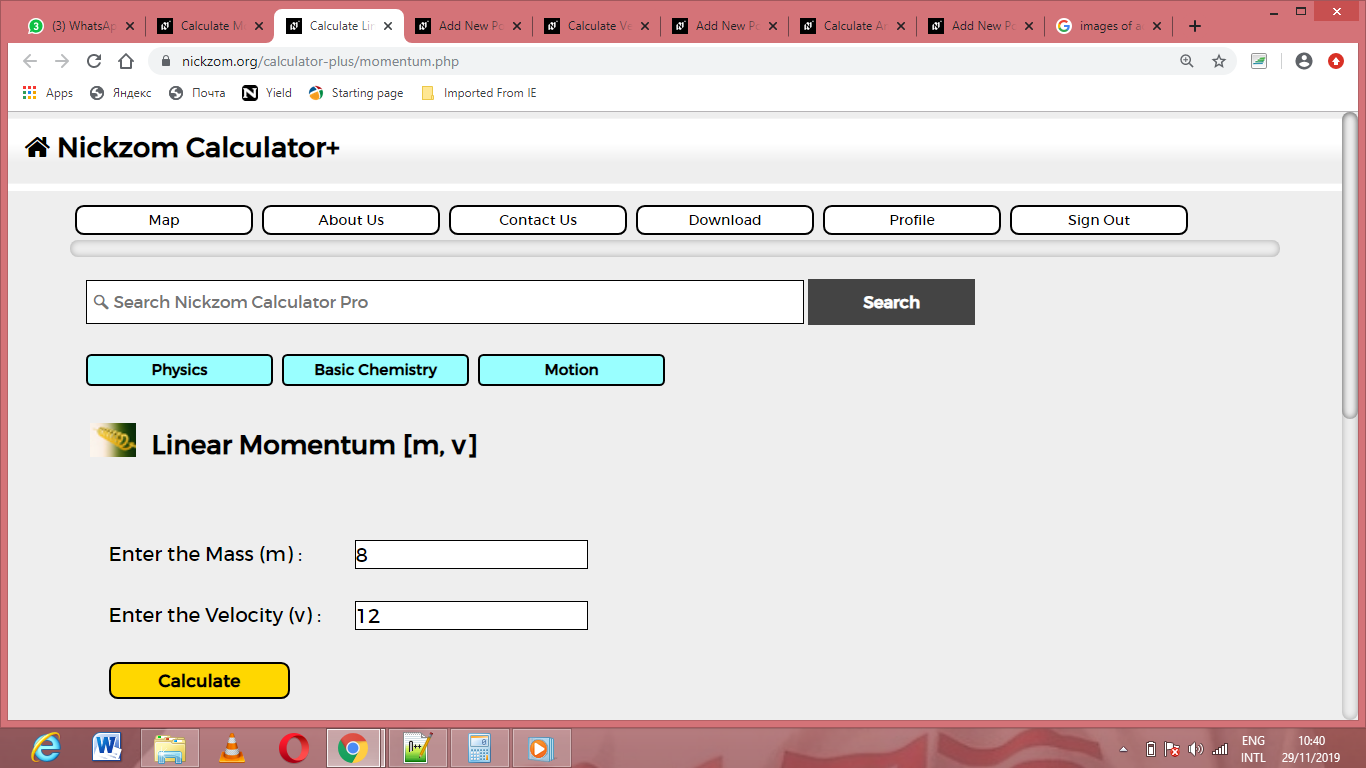Finally, Click on Calculate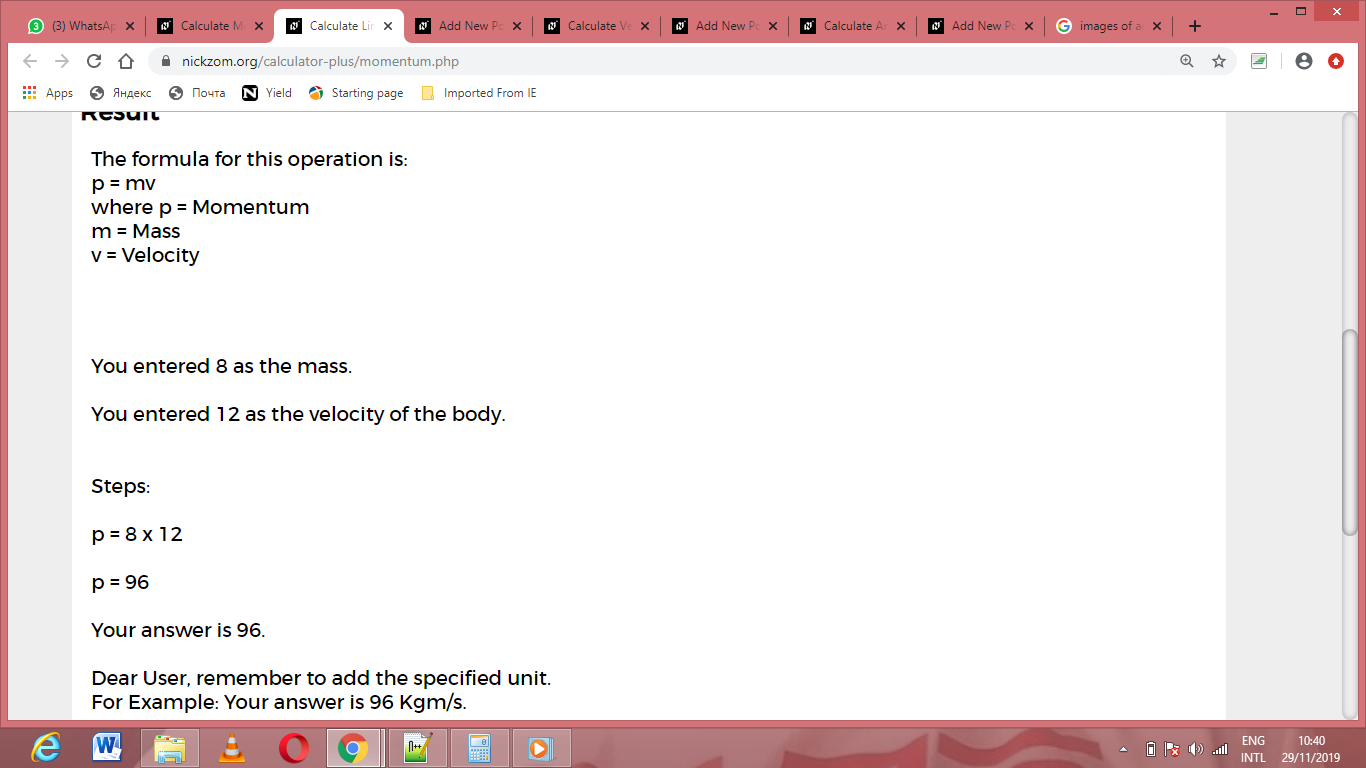As you can see from the screenshot above, Nickzom Calculator– The Calculator Encyclopedia solves for the linear momentum and presents the formula, workings and steps too.